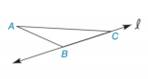Chapter 2.6, Problem 13EElementary Geometry For College St...

7th Edition
Alexander + 2 others
ISBN: 9781337614085

Solutions

Chapter
SectionElementary Geometry For College St...

7th Edition
Alexander + 2 others
ISBN: 9781337614085
Textbook Problem

Complete each figure so that it reflects across line l .To determine

a)

To draw:

The complete figure symmetrical to line 𝓁.

Explanation

Definition Used:

The figure to be reflected across the given line this implies that for every point A on the left side of the line, there should be second point A such that line should act as the perpendicular bisector of AA¯

To determine

b)

To draw:

The complete figure symmetrical to line 𝓁.

Still sussing out bartleby?

Check out a sample textbook solution.

See a sample solution

The Solution to Your Study Problems

Bartleby provides explanations to thousands of textbook problems written by our experts, many with advanced degrees!

Get Started

Find the distance between the points. 4. (1, 6), (1, 3)

Single Variable Calculus: Early Transcendentals, Volume I

Describe the scores in a sample that has standard deviation of zero.

Statistics for The Behavioral Sciences (MindTap Course List)

In Exercises 1-6, simplify the expression. 27+12

Calculus: An Applied Approach (MindTap Course List)

In Exercises 1728, use the logarithm identities to obtain the missing quantity.

Finite Mathematics and Applied Calculus (MindTap Course List)

Use sets toshow that 06=0.

Mathematical Excursions (MindTap Course List)

In problems 37-42, use technology to solve each system of equations, if a solution exists.

Mathematical Applications for the Management, Life, and Social Sciences

EXPLORING CONCEPTS f(x)=2xx2+1,[0,4] (a) 3 (b) 7 (c) -2 (d) 5 (e) 1

Calculus: Early Transcendental Functions (MindTap Course List)